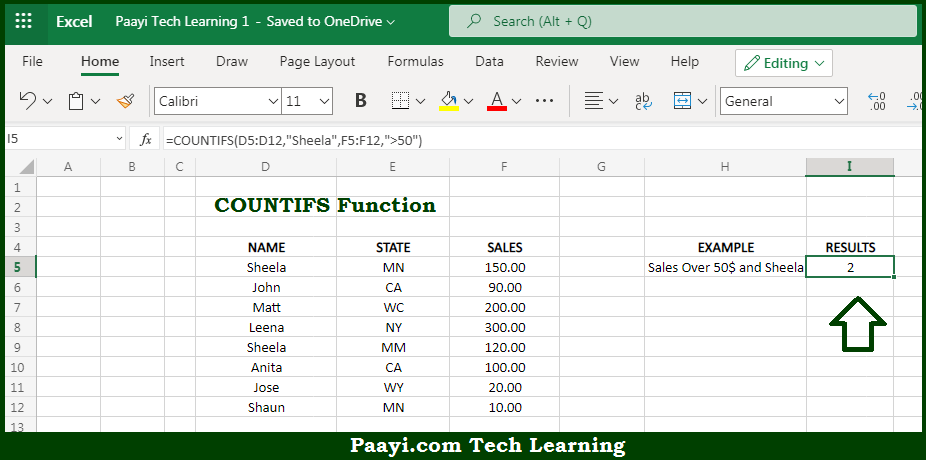# Learn How to Use Microsoft Excel COUNTIFS Function

Written by | 0 Comments | 652 Views

In this article, you will learn how to use the Microsoft Excel COUNTIFS function and its prime function in Microsoft Excel. You will also get to know the Microsoft Excel COUNTIFS function return value and syntax with the help of some examples.

Microsoft Excel COUNTIFS Function

The main purpose of the Microsoft Excel COUNTIFS function is to count the cells that match multiple criteria. That implies, with the help of the COUNTIFS function you can able to return a count of a cell that meets one or more conditions. It should be noted that the COUNTIFS function can be used to count cells based on the criteria like dates, numbers, and text. The criteria used in COUNTIFS supports logical operators (>,<,<>,=) and wildcards (*,?) for partial matching. So, with the help of the COUNTIFS function, you can able to count the cells that match multiple criteria.

Return Value of COUNTIFS Function

The return value will be the number of times the criteria is fulfilled.

Syntax of COUNTIFS Function

=COUNTIFS(range1, criteria1, [range2], [criteria2], ...)

Where the arguments:

• range1: This is the first range of cells to evaluate.
• criteria1: This is the first criteria to use on range1.
• range2: This is the second range of cells to evaluate (optional).
• criteria2: This is the second criteria to use on range2.

## How to Use Microsoft Excel COUNTIFS Function?So we know that Microsoft Excel COUNTIFS function you can able to count the cells that match multiple criteria. That implies, with the help of the COUNTIFS function you can able to return a count of a cell that meets one or more conditions. It should be noted that the COUNTIFS function can be used to count cells based on the criteria like dates, numbers, and text. The criteria used in COUNTIFS supports logical operators (>,<,<>,=) and wildcards (*,?) for partial matching. So, with the help of the COUNTIFS function, you can able to count the cells that match multiple criteria.